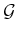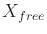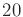#### Efficiently finding nearest points

The issues of Section 5.5.2 arise again for RDTs under differential constraints. In fact, the problem is further complicated because the edges inare generally curved. This prevents the use of simple point-segment distance tests. Furthermore, an exact representation of the state trajectory is usually not known. Instead, it is approximated numerically by the system simulator. For these reasons, it is best to use the approximate method of determining the nearest point in the swath, which is a straightforward extension of the discussion in Section 5.5.2; recall Figure 5.22. Intermediate vertices may be inserted if the applied motion primitive yields a state trajectory that travels far in. If the dimension is low enough (e.g., less than), then efficient nearest-neighbor algorithms (Section 5.5.2) can be used to offset the cost of maintaining intermediate vertices.

Steven M LaValle 2020-08-14